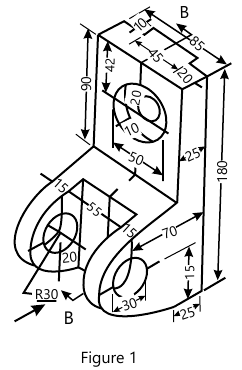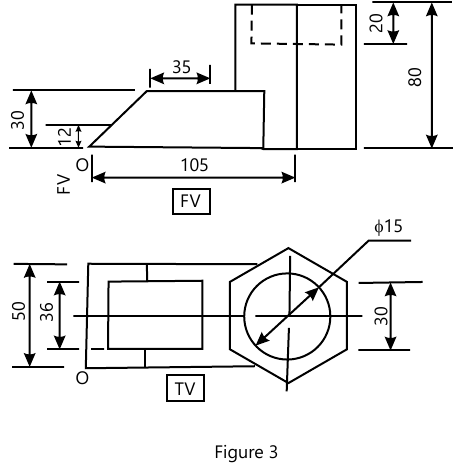MORE IN Engineering Drawing
MU First Year Engineering (Semester 2)
Engineering Drawing
May 2012
Total marks: --
Total time: --
INSTRUCTIONS
(1) Assume appropriate data and state your reasons
(2) Marks are given to the right of every question
(3) Draw neat diagrams wherever necessary

1 Figure shows pictorial view of an object. Draw
I) Front view.
II) Sectional right hand side view along section plane B-B.
III) Top view.
Dimension the views.15 M

2 A square pyramid of 30 mm edge of base and 60 mm length of axis is having an edge of base inclined at 45° to the HP. Draw the projections f the triangular face containing that edge is inclined at 30° to VP.
15 M

3 A pentagonal pyramid, base 30 mm side and axis 60 mm long rests on one of its triangular faces in HP with an edge of base perpendicular to VP. it is cut by a section plane which is perpendicular to HP and inclined at 30° to VP and bisecting the axis. Draw sectional FV, TV and true shape of section of pyramid. Also draw the development of lateral surface of uncut pyramid.
15 M

4 (a) The projections of a line PQ measures 80 mm in the TV and 70 mm in the FV. The midpoint of the line is 45 mm in front of VP and 35 mm above HP. End P is 10 mm in front of VP and nearer to it. The other end is nearer to HP. Draw the projections of the line, find the true length and true inclinations.
12 M
4 (b) Draw free hand sketch showing three views of hexagonal nut.
3 M

5 Figure shows FV and RHSV of an object. Draw
(i) Sectional FV along section plane A-A.
(ii) Sectional SV along section plane B-B.
(iii) Top view.
Dimension the views.
15 M

6 (a) Two fixed points are 100 mm apart. A point P moves in such a way that the sum of its distances from the two fixed points is always a constant and equal to 150 mm. Tace the path of the point and name the curve.
10 M
6 (b) Draw free hand sketchs of the following:-
(i) Conventional representation of internal and external threads when sectioned.